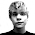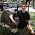## Sunday, March 2, 2014

### Boston Key Party - R3V3 writeup

Unfortunately we didn't have too many people or too much time to compete in this year's Boston Key Party. It was a very well organized CTF, with some high quality tasks. Here is my writeup for the 250 point reversing task.

## R3V3

We received a 64-bit ELF, disguised as an exe file. This is what it does when we start it:
\$ ./R3v3.exe
+  Stopped                 ./R3v3.exe
The binary was packed by UPX, so the first step was to unpack it.
\$ upx -d R3v3.exe
Then we loaded the file into IDA and looked around. There were some promising strings, that told us where to look in the code. The whole checking mechanism starts at 0x40112A. After some renaming, we found something that we have to patch in order to go forward with the solution:
.text:000000000040116A call level_2
But since we ended up solving the whole task with just static analysis, we didn't have to do that. What I named as level_2 starts at 0x4011E4. This is where the fun begins. We are asked for a password and then some form of checksum is calculated, that has to be equal to 0x936.

The checksum algorithm is not very interesting here, because we ended up solving the task without having to consider it. The reason for this, is that we get enough information about the password in the next step, which is called level_1 here. (I named them backwards, starting from 0.) Level 1 (at 0x40126A) does 3 consecutive checks, and this is the heart of the crackme.

Let's look at those check_pass functions now. I will present only one of them here, because they are very similar. check_pass_3 uses 2 different indexes to go through our password.

Both index_1 and index_1b are incremented by 1 at each turn. Index_1 indexes an array I named _weird_iv_2. I didn't know what it was for at the time, a better fitting name would be checksum_arr_2. What this function does is basically compare pairs of password[i] + password[i + 2] to predefined numbers in the checksum array. This, and all the other check_pass functions give us lots of information about the password. Here it is summarised:

• checksum_1[i] = password[i/3] + password[i/3 + 1] + password[i/3 + 2]
• checksum_2[i] = password[i] + password[i + 2]
• checksum_3[i] = password[i] + password[i + 3]
Here I was wishing that I knew how to use z3. Instead I had to solve it "by hand". It wasn't too hard, since if we guess the first character we can devise a whole 3 character block and the next character. Here is the code if anybody is interested: https://gist.github.com/balidani/596cd8cd11da0b955ff3

The resulting password was MA_SECONDO_VOI_E_STATO_ANTANI?. The final level will cut out "ANTANI" from this and display that as the key.

This was a simple but enjoyable reversing task. Thanks to the organizers for the nice CTF!

1.how to bypass .text:0000000000401165 call bad_level ? I mean der is a cmp before that which leads to bad_boy .

2.You can just patch the binary and put NOPs there instead of the call. But we ended up solving everything with static analysis.

3.Might not be the best Z3, but this was my solution for it. The output needed some manipulation (sorting, cleaning, convert from int to char). Nice work!

a, b, c, d, e, f, g, h, i, j, k, l, m, n, o, p, q, r, s, t, u, v, w, x, y, z, aa, ab, ac, ad = Ints('a b c d e f g h i j k l m n o p q r s t u v w x y z aa ab ac ad')
c_abc, c_def, c_ghi, c_jkl, c_mno, c_pqr, c_stu, c_vwx, c_yzaa, c_abacad = Bools('c_abc c_def c_ghi c_jkl c_mno c_pqr c_stu c_vwx c_yzaa c_abacad')
p1, p2, p3, p4, p5, p6, p7, p8, p9, p10, p11, p12, p13, p14, p15, p16, p17, p18, p19, p20, p21, p22, p23, p24, p25, p26, p27, p28 = Bools('p1 p2 p3 p4 p5 p6 p7 p8 p9 p10 p11 p12 p13 p14 p15 p16 p17 p18 p19 p20 p21 p22 p23 p24 p25 p26 p27 p28')

slvr = Solver()
slvr.add(Implies(p1, a + c == 0xAC),
Implies(p2, b + d == 0x94),
Implies(p3, c + e == 0xa4),
Implies(p4, d + f == 0x96),
Implies(p5, e + g == 0x94),
Implies(p6, f + h == 0x91),
Implies(p7, g + i == 0x93),
Implies(p8, h + j == 0x9d),
Implies(p9, i + k == 0xa3),
Implies(p10, j + l == 0xa5),
Implies(p11, k + m == 0xae),
Implies(p12, l + n == 0x9f),
Implies(p13, m + o == 0xae),
Implies(p14, n + p == 0x8e),
Implies(p15, o + q == 0xbe),
Implies(p16, p + r == 0x98),
Implies(p17, q + s == 0xb3),
Implies(p18, r + t == 0x94),
Implies(p19, s + u == 0xa8),
Implies(p20, t + v == 0x90),
Implies(p21, u + w == 0xb3),
Implies(p22, v + x == 0x90),
Implies(p23, w + y == 0xad),
Implies(p24, x + z == 0x95),
Implies(p25, y + aa == 0x8f),
Implies(p26, z + ab == 0xa2),
Implies(p27, aa + ac == 0x8a),
Implies(p28, ab + ad == 0x8d),
Implies(c_abc, a + b + c == 0xed),
Implies(c_def, d + e + f == 0xdb),
Implies(c_ghi, g + h + i == 0xe1),
Implies(c_jkl, j + k + l == 0x104),
Implies(c_mno, m + n + o == 0xf7),
Implies(c_pqr, p + q + r == 0xf7),
Implies(c_stu, s + t + u == 0xe9),
Implies(c_vwx, v + w + x == 0xef),
Implies(c_yzaa, y + z + aa == 0xe3),
Implies(c_abacad, ab + ac + ad == 0xd6))

#>implying

print slvr.check(p1, p2, p3, p4, p5, p6, p7, p8, p9, p10, p11, p12, p13, p14, p15, p16, p17, p18, p19, p20, p21, p22, p23, p24, p25, p26, p27, p28, c_abc, c_def, c_ghi, c_jkl, c_mno, c_pqr, c_stu, c_vwx, c_yzaa, c_abacad)
print slvr.model()

1.Very nice, thanks! Is there a way to do this with a loop in z3?

2.This was in Z3Py. You would loop through the variable creation and append them to a list. Do the same for the conditions and save them to a separate list.

Then loop through your values (0xAC, 0x94, etc.) and build all the Implies statements.

I'll see if I can whip something up like that if I have time later this week. It would have saved me some trouble during the CTF. Doing everything by hand was a bit tedious.